# Forestry workers

In the forest is employed 56 laborers planting trees in nurseries. For 8 hour work day would end job in 37 days. After 16 days, 9 laborers go forth?

How many days are needed to complete planting trees in nurseries by others, if they will work 10 hours a day?

Result

x =  20 d

#### Solution:

56 * 8 * 37 = 16 * 8 * 56 + (56 - 9) * 10 * x

470x = 9408

x = 4704235 ≈ 20.017021

Calculated by our simple equation calculator.Our examples were largely sent or created by pupils and students themselves. Therefore, we would be pleased if you could send us any errors you found, spelling mistakes, or rephasing the example. Thank you!

Leave us a comment of this math problem and its solution (i.e. if it is still somewhat unclear...):Be the first to comment!Tips to related online calculators
Need help calculate sum, simplify or multiply fractions? Try our fraction calculator.
Check out our ratio calculator.
Do you have a linear equation or system of equations and looking for its solution? Or do you have quadratic equation?
Do you want to convert time units like minutes to seconds?

## Next similar math problems:

1. Aircraft angines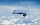The two engines of the aircraft are enough to supply the fuel for five hours of operation. However, one of the engines has a malfunction and thus consumes one-third more fuel. How long can the plane be in the air before it runs out of fuel? After an hour
2. Sum of the seventeen numbersThe sum of the 17 different natural numbers is 154. Determine the sum of the two largest ones.
3. Water current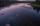John swims upstream. After a while, he passes the bottle, from that moment he floats for 20 minutes in the same direction. He then turns around and swims back, and from the first meeting with the bottle, he sails 2 kilometers before he reaches the bottle.
4. Same force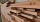The trunk of 5m length and 95 kilograms has a center of gravity 2m from the thicker end. The tribe is carried by two men, one at the thicker end. At what distance does the trunk carry a man from the other end to make the same force on it?
5. Rotaty motionWhat is the minimum speed and frequency that we need to rotate with water can in a vertical plane along a circle with a radius of 70 cm to prevent water from spilling?
6. TroopsThere are two leaders in the tourist group, with an average age of 30 years and several children with an average age of 10 years. The total age of the group is 12 years. How many children are in the group?
7. Bent scaleMonica weighed 52 kg. Sara 54 kg. Together they weighed 111 kg. They noticed that the weight on the scale was bent. How much did they really weigh?
8. School year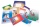At the beginning of the school year, 396 notebooks and 252 textbooks are ready to be distributed in the classroom. All pupils receive the same number of notebooks and the same amount of textbooks. How many pupils are there in the class if you know that th
9. The touristThe tourist wanted to walk the route 16 km at a specific time. He, therefore, came out at the necessary constant speed. After a 4 km walk, however, he fell unplanned into the lake, where he almost drowned. It took him 20 minutes to get to the shore and re
10. Fall sum or sameFind the probability that if you roll two dice, it will fall the sum of 10, or the same number will fall on both dice.
11. AnnulusTwo concentric circles with radii 1 and 9 surround the annular circle. This ring is inscribed with n circles that do not overlap. Determine the highest possible value of n.
12. Large familyI have as many brothers as sisters and each my brother has twice as many sisters as brothers. How many children do parents have?
13. Birth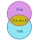Let's assume that the probability of the birth of a boy and a girl in the family is the same. What is the probability that in a family with five children, the youngest and oldest child is a boy?
14. Fast tourists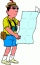If three tourists pass the route in 5 hours, how long will the same route take six equally fast tourists?
15. Magnitude of angleWhat magnitude has an obtuse angle enclosed by the hands of clocks at 12:20 hours?
16. DivideHow many different ways can three people divide 7 pears and 5 apples?
17. TV competitionIn the competition, 10 contestants answer five questions, one question per round. Anyone who answers correctly will receive as many points as the number of competitors answered incorrectly in that round. One of the contestants after the contest said: We